﻿ 连续管空气钻井排屑能力分析

# 连续管空气钻井排屑能力分析The Analysis of Cuttings Removal Ability in Air Drilling with Coiled Tubings

Abstract: In air drilling with coiled tubings, the key was to recycle broken rock particles out of the wellbore. According to the fractal theory of rock fragmentation, the shape of rock was self-similar, and the particle size conformed to the law of Weibull Distribution Law. The BWRS equation was used to calculate the air density and Lemmon method was used to calculate air viscosity and Weibull Distribution Law to fit the particle size distribution. The drag force relation of Bagheri non-spherical particles was used to calculate the air resistance of cuttings and the air velocity and pressure distribution in the wellbore, and the wellhead pressure of coiled tubing drilling. The minimum flow rate calculated with the algorithm presented in this paper is in good agreement with the flow rate in the literature. The feasibility of air drilling with coiled tubing is also proved.

1. 引言

2. 空气的物理性质

2.1. 空气的密度

$\frac{p}{\rho }=ZRT$ (1)Table 1. The comparison of air density calculated with BWRS

2.2. 空气黏度计算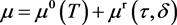(2)

${\mu }^{0}\left(T\right)=\frac{0.0266958\sqrt{MT}}{{\sigma }^{2}\Omega \left({T}^{*}\right)}$ (3)

$\Omega \left({T}^{\text{*}}\right)=\text{exp}\left(\underset{i=0}{\overset{4}{\sum }}{b}_{i}{\left[\mathrm{ln}\left({T}^{*}\right)\right]}^{i}\right)$ (4)

${\mu }^{\text{r}}\left(\tau ,\delta \right)=\underset{j=1}{\overset{n}{\sum }}{N}_{j}{\tau }^{{t}_{j}}{\delta }^{{d}_{j}}\mathrm{exp}\left(-{\gamma }_{j}{\delta }^{{l}_{j}}\right)$ (5)

3. 岩屑颗粒形状与粒径分布

3.1. 岩屑的颗粒形状

3.2. 岩屑的粒径分布

$F\left(x\right)=1-{e}^{-{\left(x/\eta \right)}^{m}}$ (6)

$\mathrm{ln}\mathrm{ln}\frac{1}{1-F\left(x\right)}=m\mathrm{ln}x-m\mathrm{ln}\eta$ (7)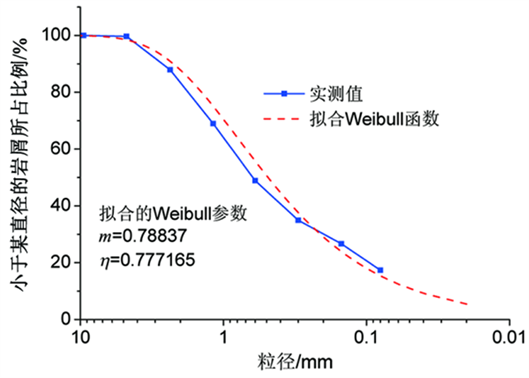Figure 1. The comparison of fitting Weibull distribution and measured values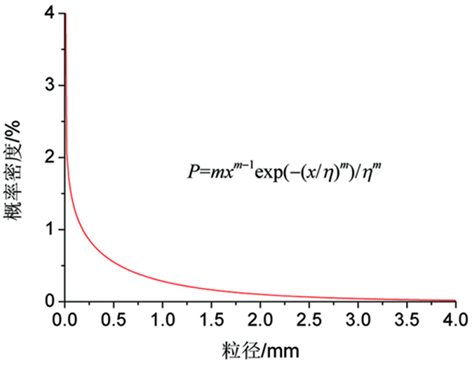Figure 2. The Weibull distribution probability density of particle size in Well Puguang 103-4

4. 岩屑颗粒在气体中的拖曳力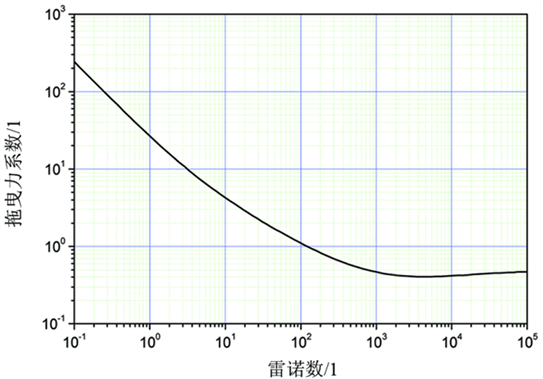Figure 3. The relationship between drag coefficient of spherical particles and Reynolds number

${C}_{D}=\frac{24{K}_{\text{S}}}{\mathrm{Re}}\left[1+0.125{\left(\mathrm{Re}{K}_{\text{N}}/{K}_{\text{S}}\right)}^{2/3}\right]+\frac{0.46{K}_{\text{N}}}{1+5330/\left(\mathrm{Re}{K}_{\text{N}}/{K}_{\text{S}}\right)}$ (8)

${K}_{\text{S}}=\left({F}_{\text{S}}^{1/3}+{F}_{\text{S}}^{-1/3}\right)/2$

${K}_{\text{N}}={10}^{{\alpha }_{2}{\left[-\mathrm{log}\left({F}_{\text{N}}\right)\right]}^{{\beta }_{2}}}$

${\alpha }_{2}=0.45+10/\mathrm{exp}\left(2.5\mathrm{lg}{\rho }^{\prime }+30\right)$

${\beta }_{2}=1-37/\mathrm{exp}\left(3\mathrm{lg}{\rho }^{\prime }+100\right)$

${F}_{\text{S}}=f{e}^{1.3}\left(\frac{{d}_{\text{eq}}^{3}}{LIS}\right)$

${F}_{\text{N}}={f}^{2}e\left(\frac{{d}_{\text{eq}}^{3}}{LIS}\right)$

${d}_{\text{eq}}=\sqrt{LIS}$

$\text{Re}=\frac{{\rho }_{\text{f}}{d}_{\text{eq}}|{u}_{\text{p}}-{u}_{\text{f}}|}{{\mu }_{\text{f}}}$

$\psi =\text{π}{d}_{\text{eq}}^{2}/S{A}_{\text{p}}$

Ap与椭球形颗粒各轴和空气流动方向的夹角有关。如图4所示是椭圆柱绕流的流体压力图，流体的流动方向是从左到右的水平方向。当椭圆柱最大迎风面积与风向呈某一角度，如逆时针偏转30˚时，计算的流体压力图表明，流体在椭圆柱表面将产生转矩，转矩的方向为顺时针，使椭圆柱转向到最大迎风面积正对着气流方向；当椭圆柱顺时针偏转30˚时，计算的压力将使椭圆柱产生逆时针的转动。不论椭圆柱呈哪个角度，转矩都会使它回到以最大迎风面迎着空气流动方向。Figure 4. The diagram of pressure of flow around an elliptic cylinder

5. 流体压力计算流程

6. 计算示例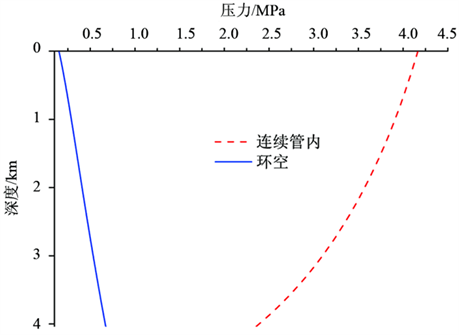Figure 5. The distribution of calculated air pressureTable 2. The calculated result of rock carrying of coiled tubing in different wellbores

7. 结论

 王立超, 范光永, 张秋菊. 空气钻井技术在伊朗项目TBK油田的应用[J]. 西部探矿工程, 2004, 16(11): 83-84.

 项德贵, 余金海, 赖晓晴, 等. 空气钻井在玉门青西油田的应用[J]. 西部探矿工程, 2006, 18(6): 178-180.

 张金成, 位华, 于文红. 空气钻井技术在普光气田的应用[J]. 石油钻采工艺, 2006, 28(6): 8-10.

 胡小房. 干空气钻井中环空携岩理论研究[J]. 石油钻井工程, 1996(2): 1-7.

 刘绘新, 孟英峰. 空气雾化钻井环空携岩流动试验研究[J]. 西南石油大学学报(自然科学版), 2001, 23(3): 34-36.

 朱红钧, 林元华, 明传中, 等. 空气携岩能力数值模拟分析[J]. 钻采工艺, 2010, 33(6): 29-30, 35.

 黄小兵, 陈次昌, 董耀文. 气体钻井的岩屑特征及粒度分布测试[J]. 天然气工业, 2008, 28(11): 83-84.

 刘毅, 周绍骑, 韩开进, 等. 基于BWRS方程的压缩空气压缩因子计算[J]. 后勤工程学院学报, 2014(4): 66-71.

 张家荣, 赵廷元. 工程常用物质的热物理性质手册[M]. 北京: 新时代出版社, 1987.

 Lemmon, E.W. and Jacobsen, R.T. (2004) Viscosity and Thermal Conductivity Equations for Nitrogen, Oxygen, Argon, and Air. International Journal of Thermophysics, 25, 21-69.
https://doi.org/10.1023/B:IJOT.0000022327.04529.f3

 巫尚蔚, 杨春和, 张超, 等. 基于Weibull模型的细粒尾矿粒径分布[J]. 重庆大学学报, 2016, 39(3): 1-12.

 Bagheri, G.H. and Bonadonna C. (2016) On the Drag of Freely Falling Non-spherical Particles. Powder Technology, 301, 526-544.
https://doi.org/10.1016/j.powtec.2016.06.015

Top